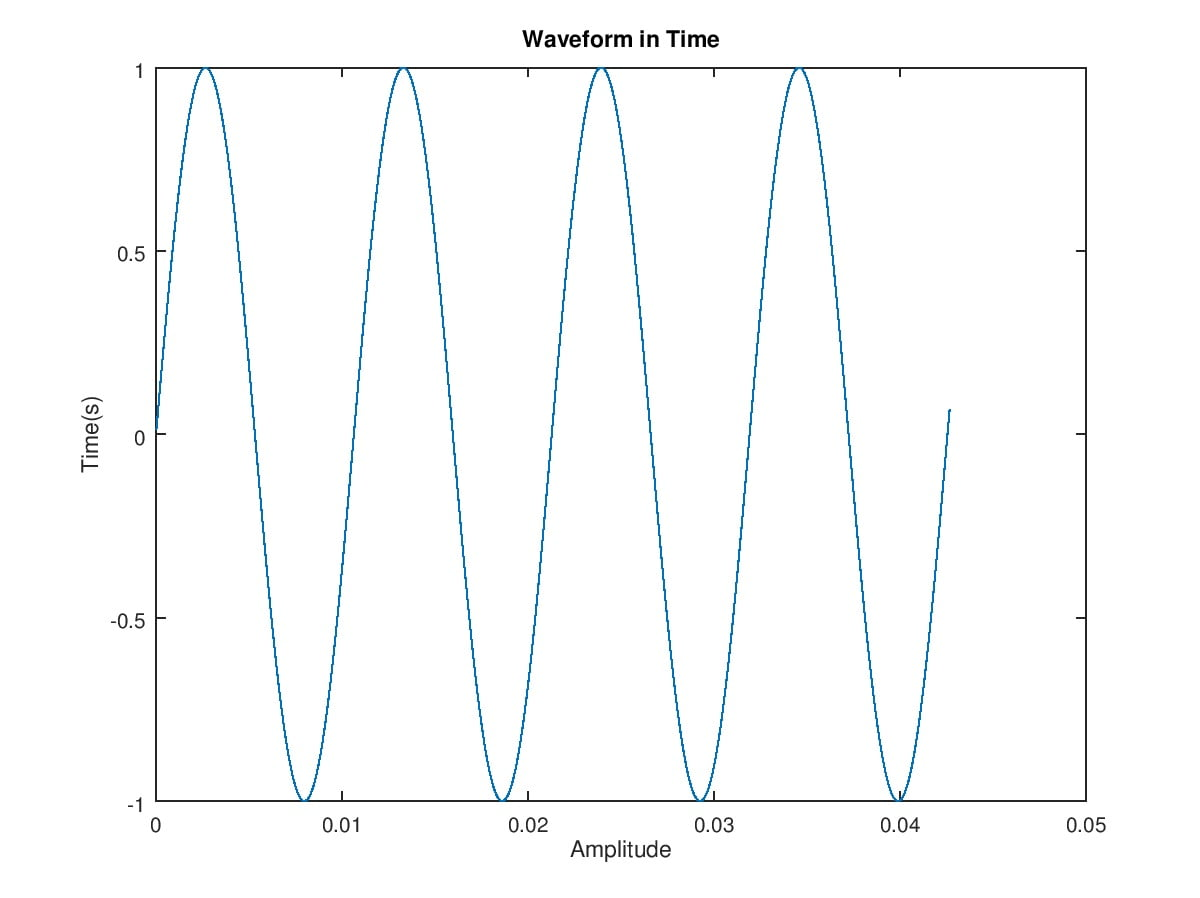# The FFT Cheat Sheet

## The FFT Cheat SheetThe FFT is a technique to determine the frequency content of a signal. FFT is an acronym for Fast Fourier Transform, where the “fast” bit simply means it is a fancy algorithm for calculating the Fourier transform, which is ultimately the calculation that takes a waveform and outputs the frequency content of the that waveform.

The waveform we input to an FFT is a set of samples. These samples would normal come from an ADC and simply be some numbers. The samples are collected at regular time intervals so we have a sample rate that is the number of samples per second ‘Fs’ and we collect a fixed number of samples ‘N’

Let take an example where the sample rate Fs is 48kHz and we collect 2048 samples (N = 2048). Now plot those samples on a graph ;

Now that looks like a sine wave and yes it is, In fact its a 94Hz sine wave. 94Hz was chosen because it happens to fit 4 sine waves perfectly into 2048 samples , sampled at 48kHz.

Next we do an FFT on the sampled data. Our FFT size will be the same as the sample size, thats is N = 2048. The FFT result gives us N (2048) complex numbers as the result.

The resulting data for our 94Hz signal looks like;

This information is not useful on it own but it does highlight one point that is for input samples that are real numbers only (i.e. no complex numbers) as is the case for all real world signals, the FFT is a mirror image of itself. So we can in fact ‘discard’ half of the data (technically speaking it represents negative frequencies) and multiply the remaining data by 2.

The FFT data is complex numbers so we will only plot the magnitude of the complex numbers i.e. FFT Magnitude = SQRT( Real(FFTData)^2 + Imag(FFTData)^2);

Each FFT number is called a bin and from 2048 samples we now get 1024 bins. The bin number can be converted to a frequency by knowing the sample rate Fs and the number of samples N. In this example each bin is spaced by Fs/N or 48000 / 2048 = 23.4Hz. To get the final frequency spectrum we then make each bin go up by 23.4Hz and plot the data with x axis as frequency in Hz

So now the graph show us that the frequency 94Hz has an average amplitude of 1 and all other frequencies are zero.

In summary;

we collect N samples at sample rate Fs
FFT the sampled data to get N FFT complex numbers
Discard half the data to get N/2 complex numbers, and multiple these numbers by 2
Calculate the bin size by Fs/N to give the frequency of each bin
Take the magnitude of the FFT data and plot against the frequency to give the FFT spectrum